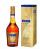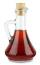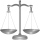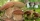# Expression of a variable from the formula + mixtures and solutions - math problems

#### Number of problems found: 11

• Solutions, mixturesHow many liters of 70% solution we must add to 5 liters of 30% solution to give us a 60% solution?
• CoinsThe money - coins are minted from the hardest bronze, which contains copper and tin in a ratio of 41: 9. How much copper and tin are in 2kg of bronze money?
• AlcoholHow many cubic centimeters of 96% alcohol is needed to obtain 0.75 liter of 40% alcohol?
• VinegarWhat percentage vinegar we get if we mix 1 if dm³ eight percent vinegar with 1.5 dm³ six percent vinegar?
• Mixture of nutsAlice bought a mixture of nuts containing cashew nuts, hazelnuts and peanuts in a ratio of 1: 2: 3. Calculate the weight in grams of the whole mixture if the peanuts weigh 90 g.
• A 36A 36 g sample of the substance contains 91% water. After drying, the weight of the sample was reduced to 18 g. What percentage of water is now in the sample?
• PeroxideHow many ml 30% of peroxide (H2O2) should be poured into 100ml H2O to give a 20% solution?
• PharmacistPharmacist had a 12 l vessel herbal lotion, which contained 30% active ingredient. Her assistant poured 18 liters of herbal lotion containing 45% of the active ingredient into a container. What percentage of herbal lotion was produced?
• Apples 2Dried apples contain 15% water. Fresh apples contain 80% water. How many kg of apples we need buy in order to get 3 kg of dried apples?
• The painterIn order for the painter to get the desired color, he must mix green and yellow in a ratio of 4: 7. If it has 28 l of green color, how many liters of yellow color should he add? How many liters of mixed color does he get?
• Sea waterMixing 62 kg of sea water with 84 kg rainwater is created water containing 3.1% salt. How many percent sea water contains salt?

We apologize, but in this category are not a lot of examples.
Do you have an interesting mathematical word problem that you can't solve it? Submit a math problem, and we can try to solve it.

We will send a solution to your e-mail address. Solved examples are also published here. Please enter the e-mail correctly and check whether you don't have a full mailbox.

Please do not submit problems from current active competitions such as Mathematical Olympiad, correspondence seminars etc...

Expression of a variable from the formula - math word problems. Mixtures and solutions - math word problems.